# The GLMSELECT Procedure

### Example 48.6 Elastic Net and External Cross Validation

This example shows how to use the elastic net method for model selection and compares it with the LASSO method. The example also uses k-fold external cross validation as a criterion in the CHOOSE= option to choose the best model based on the penalized regression fit.

This example uses a microarray data set called the leukemia (LEU) data set (Golub et al., 1999), which is used in the paper by Zou and Hastie (2005) to demonstrate the performance of the elastic net method in comparison with that of LASSO. The LEU data set consists of 7,129 genes and 72 samples, and 38 samples are used as training samples. Among the 38 training samples, 27 are type 1 leukemia (acute lymphoblastic leukemia) and 11 are type 2 leukemia (acute myeloid leukemia). The goal is to construct a diagnostic rule based on the expression level of those 7,219 genes to predict the type of leukemia. The remaining 34 samples are used as the validation data for picking the appropriate model or as the test data for testing the performance of the selected model. The training and validation data sets are available from `Sashelp.Leutrain` and `Sashelp.Leutest`.

To have a basis for comparison, use the following statements to apply the LASSO method for model selection:

```ods graphics on;
proc glmselect data=sashelp.Leutrain valdata=sashelp.Leutest
plots=coefficients;
model y = x1-x7129/
selection=LASSO(steps=120  choose=validate);
run;
```

The details about the number of observations for training and validation data are given in Output 48.6.1 and Output 48.6.2, respectively.

Output 48.6.1: Number of Training Observations Table

The GLMSELECT Procedure

Observation Profile for Analysis Data
Number of Observations Used 38
Number of Observations Used for Training 38

Output 48.6.2: Number of Validation Observations Table

Observation Profile for Validation Data
Number of Observations Used 34

Output 48.6.3 shows the "LASSO Selection Summary" table. Although the STEPS= suboption of the SELECTION= option specifies that 120 steps of LASSO selection be performed, the LASSO method terminates at step 81 because the selected model is a perfect fit and the number of effects that can be selected by LASSO is bounded by the number of training samples. Note that a VALDATA= data set is named in the PROC GLMSELECT statement and that the minimum of the validation ASE occurs at step 68. Hence the model at this step is selected, resulting in 35 selected effects. Output 48.6.4 shows the standardized coefficients of all the effects selected at some step of the LASSO method, plotted as a function of the step number.

Output 48.6.3: LASSO Selection Summary Table

 The GLMSELECT Procedure LASSO Selection Summary

Step Effect
Entered
Effect
Removed
Number
Effects In
ASE Validation
ASE
0 Intercept   1 0.8227 1.0287
1 x4847   2 0.7884 0.9787
2 x3320   3 0.7610 0.9507
3 x2020   4 0.4935 0.7061
4 x5039   5 0.2838 0.5527
5 x1249   6 0.2790 0.5518
6 x2242   7 0.2255 0.5513
. . .
. . .
. . .
62 x2534   35 0.0016 0.1554
63   x3320 34 0.0016 0.1557
64 x5631   35 0.0013 0.1532
65   x6021 34 0.0012 0.1534
66   x1745 33 0.0012 0.1535
67 x6376   34 0.0012 0.1535
68 x4831   35 0.0010 0.1531*
69   x6184 34 0.0009 0.1539
70 x3820   35 0.0006 0.1588
71 x3171   36 0.0005 0.1615
72   x6376 35 0.0004 0.1640
73 x5142   36 0.0004 0.1659
74 x1745   37 0.0003 0.1688
75 x3605   38 0.0001 0.1821
76   x573 37 0.0001 0.1825
77 x3221   38 0.0001 0.1842
78   x1745 37 0.0001 0.1842
79 x6163   38 0.0000 0.1918
80   x4831 37 0.0000 0.1920
81 x1745   38 0.0000 0.1940

Output 48.6.4: LASSO Coefficient Progression Plot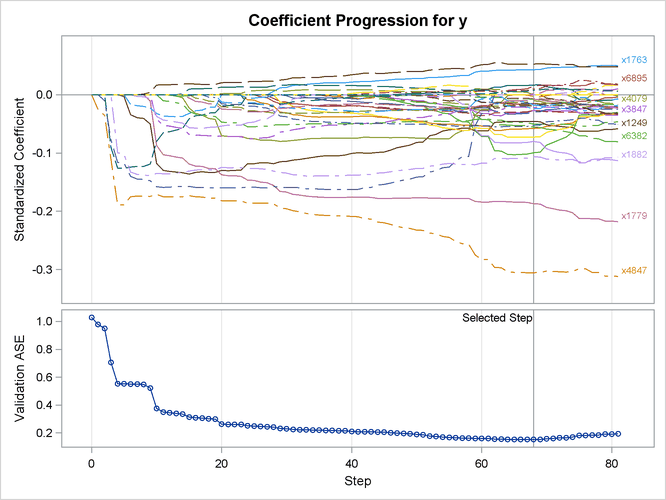As discussed earlier, the number of effects that can be selected by the LASSO method is upper-bounded by the number of training samples. However, this is not a restriction for the elastic net method, which incorporates an additional ridge regression penalty. You can use the following statements to apply the elastic net method for model selection:

```proc glmselect data=sashelp.Leutrain valdata=sashelp.Leutest
plots=coefficients;
model y = x1-x7129/
selection=elasticnet(steps=120 L2=0.001 choose=validate);
run;
```

The L2= suboption of the SELECTION= option in the MODEL statement specifies the value of the ridge regression parameter. Output 48.6.5 shows the "Elastic Net Selection Summary" table. As in the example for LASSO, the STEPS= suboption of the SELECTION= option specifies that 120 steps of elastic net selection be performed. However, in contrast to the early termination in the LASSO method at step 81, the elastic net method runs until the specified 120 steps are performed. With the same VALDATA= data set named in the PROC GLMSELECT statement as in the LASSO example, the minimum of the validation ASE occurs at step 105, and hence the model at this step is selected, resulting in 54 selected effects. Compared with the LASSO method, the elastic net method can select more variables, and the number of selected variables is not restricted by the number of samples (38 for this example). Output 48.6.6 shows the standardized coefficients of all the effects that are selected at some step of the elastic net method, plotted as a function of the step number.

Output 48.6.5: Elastic Net Selection Summary Table

 The GLMSELECT Procedure Elastic Net Selection Summary

Step Effect
Entered
Effect
Removed
Number
Effects In
ASE Validation
ASE
0 Intercept   1 0.8227 1.0287
1 x4847   2 0.7885 0.9790
2 x3320   3 0.7611 0.9510
3 x2020   4 0.4939 0.7065
4 x5039   5 0.2845 0.5533
5 x1249   6 0.2799 0.5525
6 x2242   7 0.2259 0.5517
. . .
. . .
. . .
100 x259   53 0.0000 0.1207
101 x1781   54 0.0000 0.1207
102   x7065 53 0.0000 0.1198
103 x4079   54 0.0000 0.1193
104   x6163 53 0.0000 0.1193
105 x6539   54 0.0000 0.1192*
106 x6071   55 0.0000 0.1192
107 x1829   56 0.0000 0.1192
108   x5598 55 0.0000 0.1192
109 x6169   56 0.0000 0.1192
110 x1529   57 0.0000 0.1194
111 x1306   58 0.0000 0.1194
112 x5954   59 0.0000 0.1202
113 x4271   60 0.0000 0.1203
114 x3312   61 0.0000 0.1207
115 x461   62 0.0000 0.1213
116 x4697   63 0.0000 0.1228
117   x259 62 0.0000 0.1231
118 x4186   63 0.0000 0.1252
119   x4271 62 0.0000 0.1258
120 x4939   63 0.0000 0.1261

Output 48.6.6: Elastic Net Coefficient Progression Plot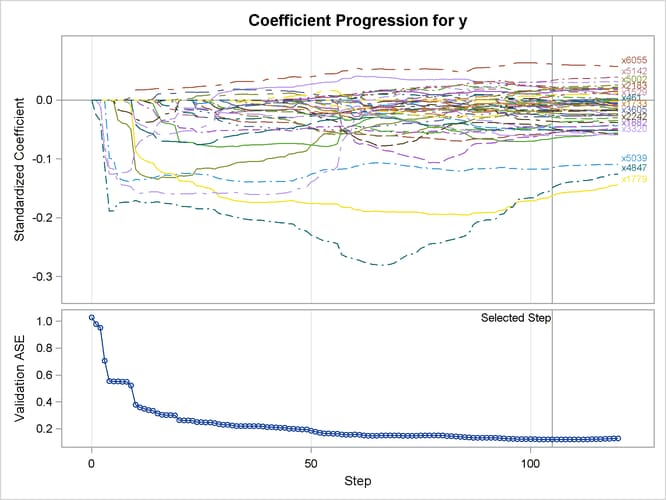When you have a good estimate of the ridge regression parameter, you can specify the L2= suboption of the SELECTION= option in the MODEL statement. However, when you do not have a good estimate of the ridge regression parameter, you can leave the L2= option unspecified. In this case, PROC GLMSELECT estimates this regression parameter based on the criterion specified in the CHOOSE= option.

If you have a validation data set, you can use the following statements to apply the elastic net method of model selection:

```proc glmselect data=sashelp.Leutrain valdata=sashelp.Leutest
plots=coefficients;
model y = x1-x7129/
selection=elasticnet(steps=120 choose=validate);
run;
```

PROC GLMSELECT tries a series of candidate values for the ridge regression parameter, which you can control by using the L2HIGH=, L2LOW=, and L2SEARCH= options. The ridge regression parameter is set to the value that achieves the minimum validation ASE (see Figure 48.12 for an illustration). Output 48.6.7 shows the "Elastic Net Selection Summary" table. Compared to Output 48.6.5, where a ridge regression parameter is specified by the L2= option, this table shows that a slightly lower validation ASE is achieved as the value of the ridge regression parameter is optimized on the validation data set.

Output 48.6.7: Elastic Net Selection Summary Table

 The GLMSELECT Procedure Elastic Net Selection Summary

Step Effect
Entered
Effect
Removed
Number
Effects In
ASE Validation
ASE
0 Intercept   1 0.8227 1.0287
1 x4847   2 0.7884 0.9787
2 x3320   3 0.7610 0.9508
3 x2020   4 0.4936 0.7061
4 x5039   5 0.2839 0.5527
5 x1249   6 0.2790 0.5519
6 x2242   7 0.2256 0.5513
. . .
. . .
. . .
110 x3605   49 0.0000 0.1252
111 x1975   50 0.0000 0.1241
112 x6757   51 0.0000 0.1224
113   x3221 50 0.0000 0.1206
114 x2183   51 0.0000 0.1205
115 x259   52 0.0000 0.1202
116 x157   53 0.0000 0.1201
117 x1781   54 0.0000 0.1197
118   x7065 53 0.0000 0.1194
119 x4079   54 0.0000 0.1187
120 x6539   55 0.0000 0.1186*

Output 48.6.8 shows the standardized coefficients of all the effects that are selected at some step of the elastic net method, plotted as a function of the step number.

Output 48.6.8: Elastic Net Coefficient Progression Plot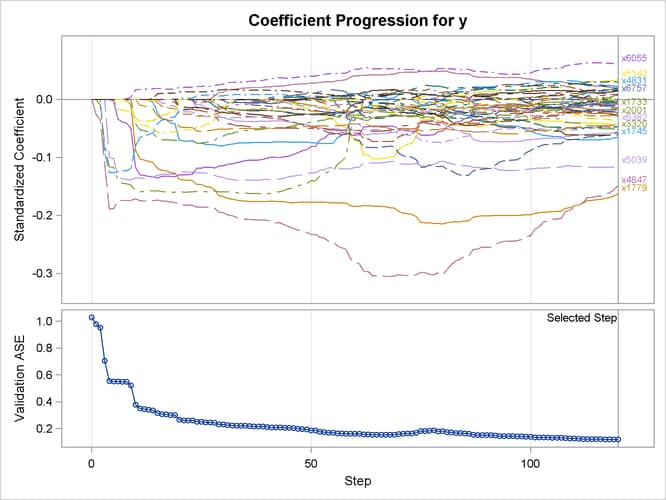When validation data are not available, you can use other criteria supported by the CHOOSE= option for estimating the value of the ridge regression parameter. Criteria such as AIC, AICC, and BIC are defined for the training samples only, and they tend to set the value of the ridge regression parameter to 0 when the number of training samples is less than the number of variables. In this case, the elastic net method reduces to the LASSO method. As an alternative to AIC, AICC, and BIC, you can use either k-fold cross validation or k-fold external cross validation in the CHOOSE= option, where the criterion is computed for held-out data.

If you want to use k-fold cross validation for selecting the model and selecting the appropriate value for the ridge regression parameter, you can use the following statements to apply the elastic net method of model selection:

```proc glmselect data=sashelp.Leutrain testdata=sashelp.Leutest
plots=coefficients;
model y = x1-x7129/
selection=elasticnet(steps=120 choose=cv)cvmethod=split(4);
run;
```

Output 48.6.9: Elastic Net Selection Summary Table

 The GLMSELECT Procedure Elastic Net Selection Summary

Step Effect
Entered
Effect
Removed
Number
Effects In
ASE Test ASE CV PRESS
0 Intercept   1 0.8227 1.0287 31.4370
1 x4847   2 0.7884 0.9787 10.6957
2 x3320   3 0.7610 0.9507 10.2065
3 x2020   4 0.4935 0.7061 7.5478
4 x5039   5 0.2838 0.5527 5.7695
5 x1249   6 0.2790 0.5518 5.0187
6 x2242   7 0.2255 0.5513 5.4050
. . . .
. . . .
. . . .
80   x4831 37 0.0000 0.1919 24.0303
81 x1745   38 0.0000 0.1933 24.0303
82 x4831   39 0.0000 0.1937 24.0303
83 x2661   40 0.0000 0.1864 24.0303
84   x3605 39 0.0000 0.1857 24.0303
85   x464 38 0.0000 0.1857 7.2724
86 x5766   39 0.0000 0.1854 7.2724
87 x464   40 0.0000 0.1763 7.2724
88 x6376   41 0.0000 0.1735 7.2724
89   x464 40 0.0000 0.1675 7.2724
90   x4079 39 0.0000 0.1674 1.2851
91 x1987   40 0.0000 0.1625 1.2846*

A TESTDATA= data set is named in the PROC GLMSELECT statement; this is the same as the validation data set used earlier. Because the L2= option is not specified, PROC GLMSELECT tries a series of candidate values for the ridge regression parameter according to the CHOOSE=CV option, and the ridge regression parameter is set to the value that achieves the minimal CVPRESS score. Output 48.6.9 shows the "Elastic Net Selection Summary" table, which corresponds to the ridge regression parameter selected by k-fold cross validation. The elastic net method achieves the smallest CVPRESS score at step 91. Hence the model at this step is selected, resulting in 40 selected effects.

Output 48.6.10 shows the standardized coefficients of all the effects selected at some step of the elastic net method, plotted as a function of the step number. Note that k-fold cross validation uses a least squares fit to compute the CVPRESS score. Thus the criterion does not directly depend on the penalized regression used in the elastic net method, and the CVPRESS curve in Output 48.6.10 has abrupt jumps.

Output 48.6.10: Elastic Net Coefficient Progression Plot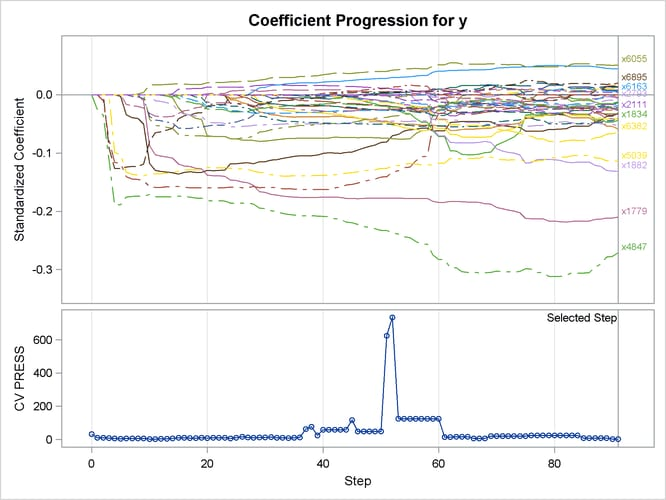If you want to perform the selection of both the ridge regression parameter and the selected variables in the model based on a criterion that directly depends on the regression used in the elastic net method, you can use k-fold external cross validation by specifying the following statements:

```proc glmselect data=sashelp.Leutrain testdata=sashelp.Leutest
plots=coefficients;
model y = x1-x7129/
selection=elasticnet(steps=120 choose=cvex)cvmethod=split(4);
run;
```

Output 48.6.11: Elastic Net Coefficient Progression Plot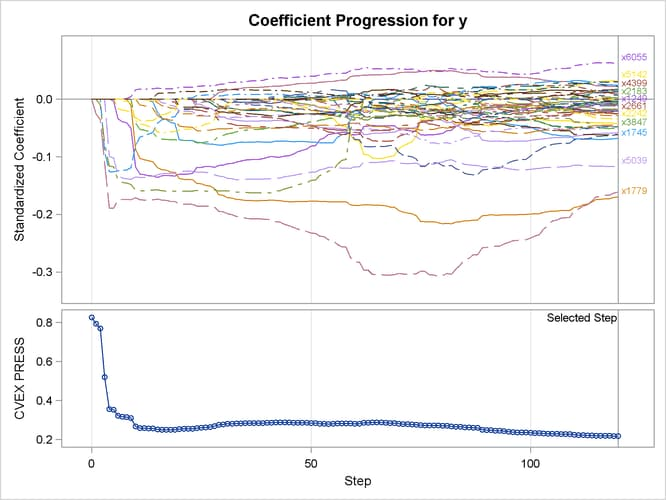As earlier, a TESTDATA= data set is named in the PROC GLMSELECT statement to test the prediction accuracy of the model on the training samples. Because the L2= option is not specified, PROC GLMSELECT tries a series of candidate values for the ridge regression parameter. Output 48.6.11 shows the standardized coefficients of all the effects selected at some step of the elastic net method, plotted as a function of the step number, and also the curve of the CVEXPRESS statistic as a function of the step number. A comparison between the criterion curves in Output 48.6.10 and Output 48.6.11 shows that the CVEXPRESS statistic is smoother than the CVPRESS statistic. Note that the CVEXPRESS statistic is based on a penalized model, whereas the CVPRESS statistic is based on an ordinary least squares model. Output 48.6.12 shows the "Elastic Net Selection Summary" table, which corresponds to the ridge regression parameter selected by k-fold external cross validation. The elastic net method achieves the smallest CVEXPRESS score at step 120, and hence the model at this step is selected, resulting in 53 selected effects.

Output 48.6.12: Elastic Net Selection Summary Table

 The GLMSELECT Procedure Elastic Net Selection Summary

Step Effect
Entered
Effect
Removed
Number
Effects In
ASE Test ASE CVEX PRESS
0 Intercept   1 0.8227 1.0287 0.8265
1 x4847   2 0.7884 0.9787 0.7940
2 x3320   3 0.7610 0.9507 0.7698
3 x2020   4 0.4936 0.7061 0.5195
4 x5039   5 0.2839 0.5527 0.3554
5 x1249   6 0.2790 0.5519 0.3526
6 x2242   7 0.2255 0.5513 0.3218
7 x6539   8 0.2161 0.5498 0.3166
8 x6055   9 0.2128 0.5488 0.3148
9 x1779   10 0.1968 0.5205 0.3096
10 x3847   11 0.1065 0.3765 0.2671
11 x4951   12 0.0891 0.3498 0.2586
12 x1834   13 0.0868 0.3458 0.2579
13 x1745   14 0.0837 0.3397 0.2570
14 x6021   15 0.0809 0.3355 0.2561
15 x6362   16 0.0667 0.3144 0.2510
16 x2238   17 0.0621 0.3075 0.2498
. . . .
. . . .
. . . .
100 x2121   45 0.0000 0.1402 0.2350
101 x6021   46 0.0000 0.1386 0.2334
102   x3171 45 0.0000 0.1377 0.2324
103 x4461   46 0.0000 0.1350 0.2302
104 x4697   47 0.0000 0.1338 0.2299
105   x3605 46 0.0000 0.1331 0.2293
106 x3221   47 0.0000 0.1330 0.2289
107 x5002   48 0.0000 0.1320 0.2282
108   x3820 47 0.0000 0.1319 0.2281
109   x4697 46 0.0000 0.1315 0.2278
110 x4052   47 0.0000 0.1276 0.2235
111 x6184   48 0.0000 0.1266 0.2229
112 x3605   49 0.0000 0.1252 0.2215
113 x1975   50 0.0000 0.1240 0.2212
114 x6757   51 0.0000 0.1222 0.2200
115   x3221 50 0.0000 0.1206 0.2185
116 x2183   51 0.0000 0.1205 0.2185
117 x259   52 0.0000 0.1202 0.2182
118 x157   53 0.0000 0.1201 0.2181
119 x1781   54 0.0000 0.1197 0.2177
120   x7065 53 0.0000 0.1194 0.2177*